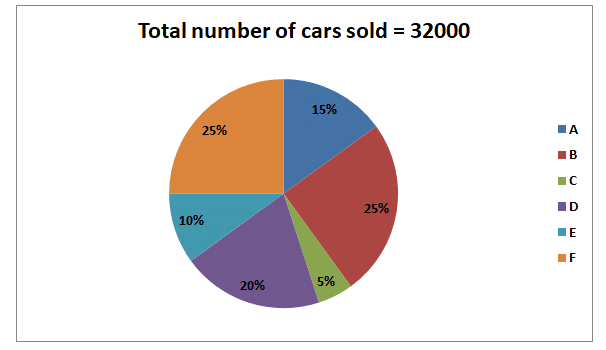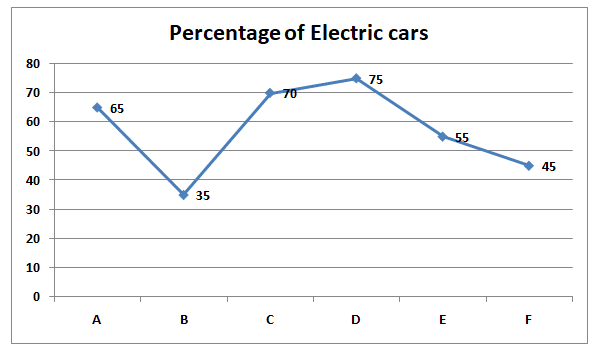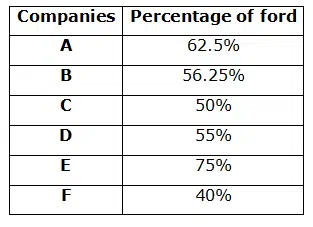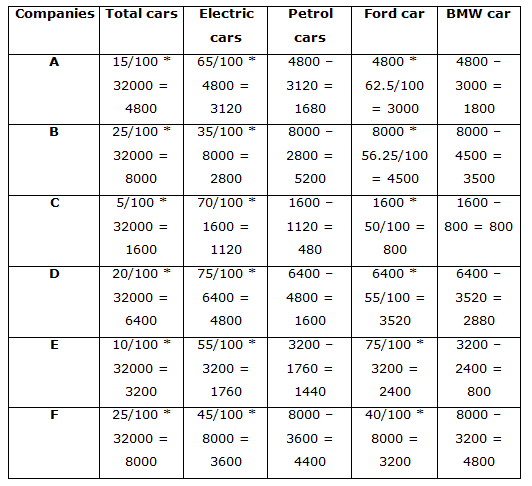# “The Knowledge Library”

Knowledge for All, without Barriers…

# “The Knowledge Library”

Knowledge for All, without Barriers……….

# DI & Caselet-1

Directions (1-5): Study the following information carefully and answer the questions given below.

The give pie chart shows the percentage distribution of the total number of cars sold by six different companies.The given line graph shows the percentage of electric cars sold by different shops.The given table shows the percent of Ford cars sold by different shops.Note: Total cars = Electric car + Petrol car = Ford + BMW

1) Ratio of the number of electric ford to electric BMW car sold by B is 9:11 and the ratio of the number of electric ford to electric BMW car sold by F is 13:7, then what is the difference between the total number of petrol BMW car sold by B and F?

A.1520

B.1540

C.1510

D.1580

E.1560

2) The sum of the total number of electric cars and the total number of petrol cars sold by F is what percent of the sum of the total number of ford cars and BMW cars sold by D?

A.120%

B.115%

C.125%

D.110%

E.130%

3) If the total number of petrol Ford car to electric Ford car sold by A in the ratio of 3:7, then the total number of Electric BMW cars sold by A is what percent of the total number of Ford car sold by C?

A.128%

B.127.5%

C.132.80%

D.122.83%

E.98%

4) What is the sum of the number of electric cars sold by A, the total number of petrol car sold by C, total number of ford car sold by D and the total number of BMW car sold by E?

A.7920

B.8040

C.7860

D.7420

E.8120

5) What is the difference between the averages of total number of Electric cars sold by A, B, C and D together and the average number of BMW cars sold by C, D, E and F together?

A.635

B.640

C.645

D.650

E.655

Caselet

Directions (06-10): Study the following information carefully and answer the questions given below.

A long route bus is driven by five drivers Anil, Buvanesh, Sita, Diya and Ezhanth one by one. The total time taken in the journey by all the drivers together is 160 hours. Ratio of the time taken by Anil to Ezhanth is 2:3 and total time by Sita is one-eighth of the total time taken in the journey. The distance covers by Anil is 840 km. The ratio of the distance covered by Anil to Ezhanth 7:9. Time taken by Buvanesh is 40% less than the time taken by Diya and the time taken by Buvanesh 10 hours more than the time taken by Sita. The distance covered by Sita is 580 km less than the distance covered by Ezhanth.

6) If the average speed of bus driving by Buvanesh is 20 kmph, then find the total distance covered by Buvanesh?

A.450 km

B.500 km

C.550 km

D.600 km

E.750 km

7) If the total journey of bus is 3320 km and the ratio of the distance travelled by Buvanesh to Diya is 4:5, then what is the speed of Diya driving bus?

A.15 kmph

B.20 kmph

C.30 kmph

D.25 kmph

E.None of these

8) If the speed of Buvanesh and Diya in the ratio of 6:7 and the difference between the speed of Buvanesh and Diya is 6 kmph, then what is the average speed of the bus driving whole journey?

A.33 kmph

B.35 kmph

C.36 kmph

D.38 kmph

E.40 kmph

9) If the distance covered by Diya is 900 km, then what is the ratio of the speed of Diya to speed of Sita?

A.3:4

B.2:3

C.9:13

D.11:18

E.18:25

10) Find the ratio of the speed of bus when Anil is driving to the speed of bus when Ezhanth driving the bus?

A.6:5

B.7:6

C.5:4

D.3:4

E.3:2

Directions (1-5) :Total number of electric sold by B = 2800

Number of electric BMW car sold by B = 2800 * 11/20 = 1540

Number of BMW petrol car sold by B = 3500 – 1540 = 1960

Total number of electric car sold by F = 3600

Number of electric BMW car sold by F = 3600 * 7/20 = 1260

Total number of BMW petrol car sold by F = 4800 – 1260 = 3540

Required difference = 3540 – 1960 = 1580

Required percentage = (4400 + 3600)/(3520 + 2880)

= (8000/6400) * 100

= 125%

Number of electric ford car sold by A = 3000 * 7/10 = 2100

Number of electric BMW car sold by A = 3120 – 2100= 1020

Required percentage = 1020/800 * 100 = 127.5%

Required sum = 3120 + 480 + 3520 + 800

= 7920

Average of total number of electric cars sold by A, B, C and D = (3120 + 2800 + 1120 + 4800)/4 = 2960

Average total number of BMW car sold by C, D, E and F = (800 + 2880 + 800 + 4800)/4

= 2320

Directions (6-10) :

Difference = 2960 – 2320 = 640

Time taken by Sita = 1/8 * 160 = 20 hours

Time taken by Buvanesh = 10 + 20 = 30 hours

Time taken by Diya = 100/60 * 30 = 50 hours

Time taken by Anil = 2x

Time taken by Ezhanth = 3x

2x + 3x + 20 + 30 + 50 = 160

5x = 60

x = 12

Time taken by Anil = 2 * 12 = 24 hours

Time taken by Ezhanth = 3 * 12 = 36 hours

Distance covered by Anil = 840 km

Speed of Anil = 840/24 = 35 kmph

Distance covered by Ezhanth = 9/7 * 840 = 1080

Speed of Ezhanth = 1080/36 = 30 kmph

Distance covered by sita = 1080 – 580 = 500 km

Speed of Sita = 500/20 = 25 kmph

Distance covered by Buvanesh = 30 * 20 = 600 km

Distance covered by Bhuvanesh and Diya = 3320 – (840 + 1080 + 500) = 900

Distance covered by Diya = 900 * 5/9 = 500 km

Required speed = 500/50 = 10 kmph

Speed of Bhuvanesh = 6 * 6 = 36 kmph

Speed of Diya = 7 * 6 = 42 kmph

Distance covered by Buvanesh = 36 * 30 = 1080

Distance covered by Diya = 42 * 50 = 2100

Required speed = (840 + 1080 + 1080 + 2100 + 500)/160

= 35kmph

Required ratio = (900/50):25

= 18:25

Required ratio = 35:30 = 7:6